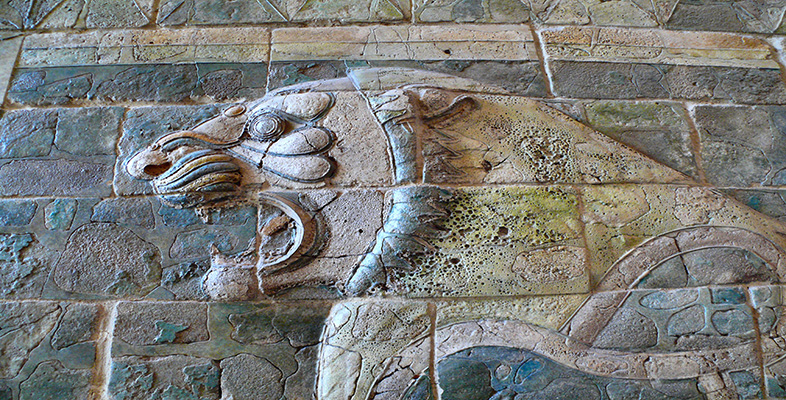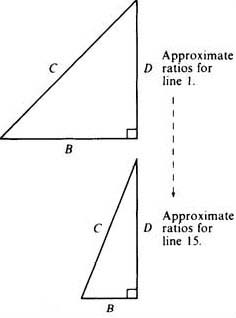Babylonian mathematics

Start this free course now. Just create an account and sign in. Enrol and complete the course for a free statement of participation or digital badge if available.

Free course

# 1.5.2 What is the significance of the numbers?

In seeking the significance of these numbers, there is more information on the tablet that we have not yet taken into account, namely the text of the column headings themselves. The heading of column A is partly destroyed, but the text headings for B and C are clearer. B says something like ‘ib-sa of the front’, and C ‘ib-sa of the diagonal’, where ib-sa is a Sumerian word whose significance here is not precisely known. The geometrical cue provided by the headings suggests that we try viewing the numbers on the tablet as relating to geometrical figures. Indeed, as the numbers in the table above have the property B2+D2=C2 (which follows immediately from the way D was defined), it appears that the middle two columns of Plimpton 322 contain the shortest side and diagonal respectively of right-angled triangles, whose third side is a simple regular number. Successive lines in the table can then be seen to be ordered so that the triangles gradually change shape from B being almost equal to C, to it being rather shorter. (This is equivalent to what is shown in column A, where the ratio C2/D2 diminishes as one goes down the column—note that the actual numerical size of the triangles changes randomly, it is just the shape that varies in a smooth ordered way.)

We should think carefully about what these geometrical connotations of the numbers on the tablet might mean. We know from several other tablets that the Babylonians were well aware that adding the squares of the lengths of the two shorter sides of a right-angled triangle gives the same number as squaring the length of the diagonal (one version of the result that has come to be called, for no very sound historical reason, ‘Pythagoras' Theorem’). But why were whole number examples of such triangles listed on this tablet? (If that is indeed what the numbers signify.) Why ordered in this curious way? Why these particular triangles? What is the purpose of column A? The successful decipherment of Plimpton 322 has managed to raise rather more questions than when it was safely catalogued as a ‘commercial account’!

Historians have reached various conclusions about what this tablet signifies. Neugebauer and Sachs, the original decipherers, regarded it as ‘the oldest preserved document in ancient number theory’ and that it concerned ‘the fundamental laws of the numbers themselves’. (O. Neugebauer and A. Sachs, Mathematical Cuneiform Texts (American Oriental Society, 1945) pp. 37, 41.) So, on this interpretation, it has little to do with geometry as such, except incidentally, but more to do with properties of numbers. For what struck them especially, when trying to work out how the table had been constructed, was that each line can arise from a pair of small regular numbers (this is the significance of the columns labelled p and q in the table on the previous page). In fact,

B = p2 − q2;   C = p2 + q2;   D = 2pq,

as you can see by trying this out for yourself on any of the lines of the above table. So it is possible (no less, no more) that the Babylonian scribe was aware of these formulae though he would not, of course, have written them in anything like the modern algebraic form just given. These formulae generate Pythagorean triples, i.e. sets of whole numbers B, D, C, such that B2 + D2=C2. Was Plimpton 322 part of an investigation of number relationships?

It is evident, certainly, that the numbers on Plimpton 322 were the result of some mathematical procedure carried out off-stage, on another tablet perhaps. Apart from common-sense grounds, this can be inferred from the nature and occurrence of the errors on the tablet.Figure 5

## Question 4

Look again at the original translation of Plimpton 322, noting where the errors by the scribe were made. Are these compatible with column A having been calculated directly from columns B and C, as our ‘formula’ A=C2/(C2B2) might have tended to suggest?

### Discussion

If column A had been calculated directly from columns B and C, then any mistake in B or C wouid carry over to A. But this has not happened, so A must have been calculated independently of B and C.

It is also worth noting that the scribe made four errors in computing the relatively simple B and C numbers, but none of consequence in the more complicated A column (there were in fact two minor confusions of place value, here ‘corrected’ in the transcription). As this is contrary to what one would expect, it seems a significant observation about the computations that went in to the tablet, even if it is not clear just what the significance is.

MA290_2